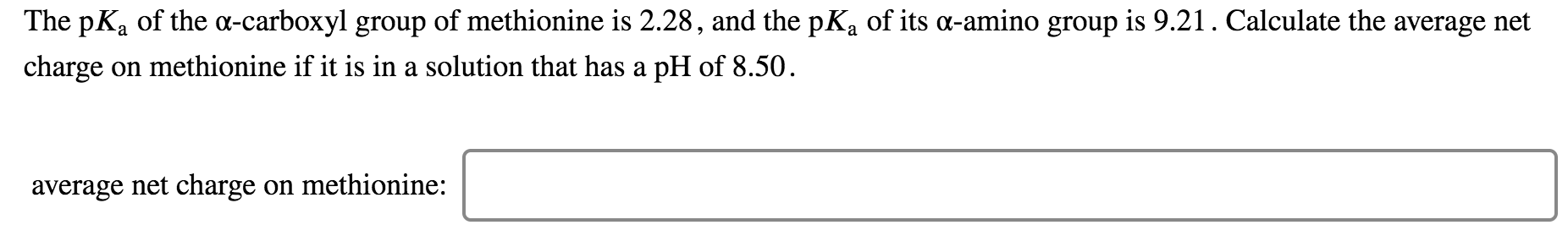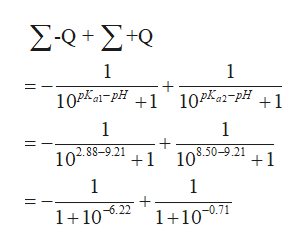# The pKa of the a-carboxyl group of methionine is 2.28, and the pKa of its a-amino group is 9.21. Calculate the average netcharge on methionine if it is in a solution that has a pH of 8.50average net charge on methionine:

Question
3 views

How is this question begun?  How is the HH equation used?help_outlineImage TranscriptioncloseThe pKa of the a-carboxyl group of methionine is 2.28, and the pKa of its a-amino group is 9.21. Calculate the average net charge on methionine if it is in a solution that has a pH of 8.50 average net charge on methionine: fullscreen
check_circle

Step 1

The Henderson-Hasselbalch equation is as follows

Step 2

On reaaranging the equation, we get the following equation

Step 3

Now when we calculate the charge on methionine...help_outlineImage TranscriptioncloseΣοΣο 1 10Pa1pH +1 10P2-pH 1 1 1 8.50-9.21 +1 102.88-9.21 1 1 11022 1+10-0.71 fullscreen

### Want to see the full answer?

See Solution

#### Want to see this answer and more?

Solutions are written by subject experts who are available 24/7. Questions are typically answered within 1 hour.*

See Solution
*Response times may vary by subject and question.
Tagged in